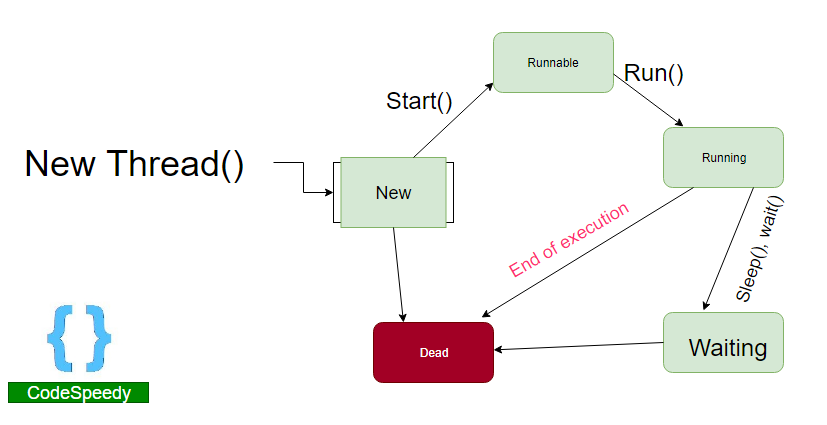We will learn the following in the following tutorial

Threads are a part of a process, while the process is being executed. Every process has at least one thread, which is the ‘Main Thread’. It is created by the Java Virtual Machine(JVM) at the program’s start, when the main() method is invoked.

## What is Multithreading in Java

Multithreading is a Java feature that allows more than one thread to run simultaneously from a single program(process). This ensures the maximum utilisation of the CPU. So, threads are light-weight processes within a process.

There are two methods to create a thread –
2. Implement the Runnable Interface.We create a class and that class must extend java.lang.Thread class. Then, we override the run() method. This method is the entry point for the thread(the life cycle begins). To call the run() method, we first create an object of the class and then call the start() method. The start() method invokes the run() method automatically, on the Thread object that is created.

Here is an example program –

```class Multithreading extends Thread
{

public void run()
{
try{
}
catch(Exception e)
{
e.printStackTrace();
}
}
}
{
public static void main(String[] args)
{
int n = 10;
for(int i=0;i<n;i++)
{
mlt.start();
}
}
```

Output –

```Current thread is: Thread-1

Note – Notice that the order might not what you expect it to. It might be different every time you run the program. The order purely depends upon how the CPU schedules the threads, and the order of scheduling is unknown to us.

### Thread creation by implementing the Runnable Interface in Java

We create a class and that class must implement java.lang.Runnable class. We override the run() method and then we instantiate a Thread object. Then, we call the start() method.

```class Multithreading implements Runnable
{

public void run()
{
try{
}
catch(Exception e)
{
e.printStackTrace();
}
}
}
{
public static void main(String[] args)
{
int n = 10;
for(int i=0;i<n;i++)
{
t.start();
}
}
}```

Output –

```Current thread is: Thread-1